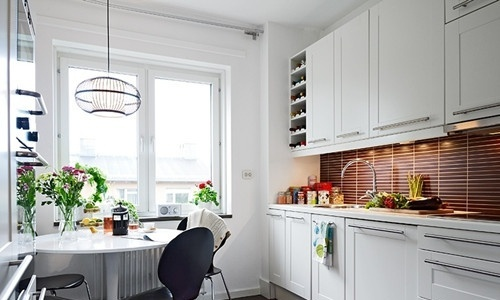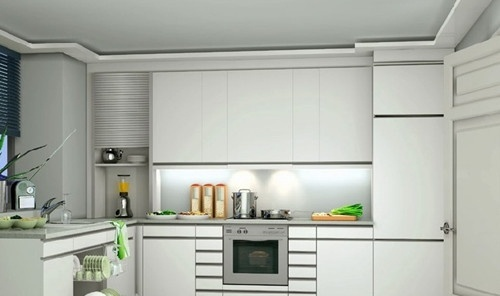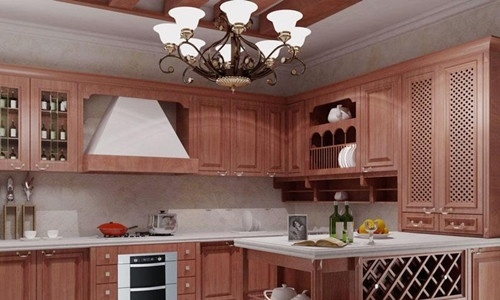|

# 品牌橱柜 品牌橱柜有哪些?

橱柜品牌有很多，国内的、国外的橱柜品牌充斥着整个橱柜市场，但橱柜品牌中能称得上品牌橱柜的就要属十大品牌了，今天就随小编来看看橱柜市场中的品牌橱柜都有哪些吧。品牌橱柜之欧派橱柜OPPEIN

欧派品牌在国内可谓是家喻户晓,拥有很高的知名度,创建于1994年的欧派是率先将欧洲整体厨房概念引入中国,将国际先进的生产工艺与中国厨房文化融合升华。多年来一直引领着中国整体橱柜行业的发展。

品牌橱柜之科宝博洛尼橱柜BOLONI

成立于1999年的科宝博洛尼橱柜,将意大利先进的厨房文化带入中国,倡导”Living in Kitchen”(生活在厨房)的崭新理念和全新生活方式,在国内掀起了一场厨房革命,结合中国人的生活习惯与使用方法,在中国橱柜市场上占据重要位置。品牌橱柜之司米橱柜SCHMIDT

Schmidt产品包括整体橱柜、浴柜、储物家具和桌椅等，并提供一站式家居设计、布局、安装的个性化专业咨询服务，为每一位追求自我表达的都市新贵提供个性化的专属定制产品，创造浪漫、自由、富有创造力的法式生活艺术。司米橱柜以打造中国家庭满意度最高的顶尖整体厨房空间为目标,引入法国先进技术,工艺流程,搭建橱柜柔性化生产系统,将执着于细节的生活态度与精致浪漫的法式优雅植入中国厨房,为中国家庭带来高品质的家居生活。

品牌橱柜之金牌橱柜GOLDENHOME

全国工商联家具装饰业商会厨柜专委会执行会长单位,中国建筑装饰协会厨卫工程委员会执行会长单位。金牌厨柜一直以来,都是面向热爱生活、积极进取的人们,让每一位使用金牌厨柜的的人都能轻松烹饪生活滋味。金牌厨柜卖的不仅仅是厨柜,而是回归生活的理念。

品牌橱柜之皮阿诺橱柜PIANO

皮阿诺科学艺术厨房,中国橱柜市场消费者满意品牌,国家奥林匹克体育中心专用产品,全国工商联橱柜专业委员会副会长单位,亚洲一流的橱柜生产企业之一。多年来，皮阿诺始终关注中国家庭的厨房需求，依托自主研发、坚持原创设计，每年都为终端奉献数套经典设计，“香雪”系列更开启了行业印花烤漆的新纪元。品牌橱柜之东方邦太

东方邦太橱柜成立于2003年,是国内著名的橱柜产品制造基地；集产品研发、生产、销售和服务为一体的专业化橱柜企业，有实木系列、压膜、烤漆系列三个系列产品。“东方”代表品牌的国别定位,“邦太”则代表民族礼仪,目标要做具有中国特色、体现全民族风格的整体橱柜。

品牌橱柜之我乐OLOKITCHEN

我乐厨柜作为中国建厂最早、规模最大的橱柜制造商之一。致力于帮助消费者构筑优质的厨房生活,并以高性价比的产品和服务赢得了客户的认可,取得了稳健地发展和巨大的市场成功。我乐厨柜作为中国整体厨柜行业的领先品牌，洞悉客户需求，创造客户价值。拥有世界至大的厨柜生产基地，引进IMA全自动生产线。独家环保承诺、超强厨柜内芯，我乐专注于构筑健康时尚的厨房生活，将欧洲设计和德国工艺完美结合。

品牌橱柜之柯拉尼KOLANI

柯拉尼传承了德国工业文明精髓,崇尚自由、自然、健康的生活方式,是纯德式生活理念的倡导者和传播者,纯德式定制家居生活方式的提供者。品牌橱柜之佳居乐CACAR

佳居乐橱柜凭借着多年来积聚的强大的规模和实力,获得国家及行业颁发的多项殊荣,在一支富有创造力与凝聚力的精英团队及优秀的销售系统平台下,一直保持着健康、稳定、快速的增长。

品牌橱柜之好兆头橱柜

迄今为止，好兆头橱柜已覆盖全国一二级城市，拥有近百个高端精装项目和国际先进的全套电子数控生产线。同时，好兆头橱柜年产值超过10万套的规模化定制专业橱柜生产基地，已成为国内最完整的厨柜产业基地。

关于品牌橱柜的相关信息就为大家介绍到这里了，希望这篇文章对大家有所帮助。如果大家还有什么不明白的地方可以在下方给小编留言哦，我们会尽快为您解答。

`声明：本文由入驻焦点开放平台的作者撰写，除焦点官方账号外，观点仅代表作者本人，不代表焦点立场错误信息举报电话： 400-099-0099，邮箱：jubao@vip.sohu.com，或点此进行意见反馈，或点此进行举报投诉。`A B C D E F G H J K L M N P Q R S T W X Y Z
A - B - C - D - E
• A
• 鞍山
• 安庆
• 安阳
• 安顺
• 安康
• 澳门
• B
• 北京
• 保定
• 包头
• 巴彦淖尔
• 本溪
• 蚌埠
• 亳州
• 滨州
• 北海
• 百色
• 巴中
• 毕节
• 保山
• 宝鸡
• 白银
• 巴州
• C
• 承德
• 沧州
• 长治
• 赤峰
• 朝阳
• 长春
• 常州
• 滁州
• 池州
• 长沙
• 常德
• 郴州
• 潮州
• 崇左
• 重庆
• 成都
• 楚雄
• 昌都
• 慈溪
• 常熟
• D
• 大同
• 大连
• 丹东
• 大庆
• 东营
• 德州
• 东莞
• 德阳
• 达州
• 大理
• 德宏
• 定西
• 儋州
• 东平
• E
• 鄂尔多斯
• 鄂州
• 恩施
F - G - H - I - J
• F
• 抚顺
• 阜新
• 阜阳
• 福州
• 抚州
• 佛山
• 防城港
• G
• 赣州
• 广州
• 桂林
• 贵港
• 广元
• 广安
• 贵阳
• 固原
• H
• 邯郸
• 衡水
• 呼和浩特
• 呼伦贝尔
• 葫芦岛
• 哈尔滨
• 黑河
• 淮安
• 杭州
• 湖州
• 合肥
• 淮南
• 淮北
• 黄山
• 菏泽
• 鹤壁
• 黄石
• 黄冈
• 衡阳
• 怀化
• 惠州
• 河源
• 贺州
• 河池
• 海口
• 红河
• 汉中
• 海东
• 怀来
• I
• J
• 晋中
• 锦州
• 吉林
• 鸡西
• 佳木斯
• 嘉兴
• 金华
• 景德镇
• 九江
• 吉安
• 济南
• 济宁
• 焦作
• 荆门
• 荆州
• 江门
• 揭阳
• 金昌
• 酒泉
• 嘉峪关
K - L - M - N - P
• K
• 开封
• 昆明
• 昆山
• L
• 廊坊
• 临汾
• 辽阳
• 连云港
• 丽水
• 六安
• 龙岩
• 莱芜
• 临沂
• 聊城
• 洛阳
• 漯河
• 娄底
• 柳州
• 来宾
• 泸州
• 乐山
• 六盘水
• 丽江
• 临沧
• 拉萨
• 林芝
• 兰州
• 陇南
• M
• 牡丹江
• 马鞍山
• 茂名
• 梅州
• 绵阳
• 眉山
• N
• 南京
• 南通
• 宁波
• 南平
• 宁德
• 南昌
• 南阳
• 南宁
• 内江
• 南充
• P
• 盘锦
• 莆田
• 平顶山
• 濮阳
• 攀枝花
• 普洱
• 平凉
Q - R - S - T - W
• Q
• 秦皇岛
• 齐齐哈尔
• 衢州
• 泉州
• 青岛
• 清远
• 钦州
• 黔南
• 曲靖
• 庆阳
• R
• 日照
• 日喀则
• S
• 石家庄
• 沈阳
• 双鸭山
• 绥化
• 上海
• 苏州
• 宿迁
• 绍兴
• 宿州
• 三明
• 上饶
• 三门峡
• 商丘
• 十堰
• 随州
• 邵阳
• 韶关
• 深圳
• 汕头
• 汕尾
• 三亚
• 三沙
• 遂宁
• 山南
• 商洛
• 石嘴山
• T
• 天津
• 唐山
• 太原
• 通辽
• 铁岭
• 泰州
• 台州
• 铜陵
• 泰安
• 铜仁
• 铜川
• 天水
• 天门
• W
• 乌海
• 乌兰察布
• 无锡
• 温州
• 芜湖
• 潍坊
• 威海
• 武汉
• 梧州
• 渭南
• 武威
• 吴忠
• 乌鲁木齐
X - Y - Z
• X
• 邢台
• 徐州
• 宣城
• 厦门
• 新乡
• 许昌
• 信阳
• 襄阳
• 孝感
• 咸宁
• 湘潭
• 湘西
• 西双版纳
• 西安
• 咸阳
• 西宁
• 仙桃
• 西昌
• Y
• 运城
• 营口
• 盐城
• 扬州
• 鹰潭
• 宜春
• 烟台
• 宜昌
• 岳阳
• 益阳
• 永州
• 阳江
• 云浮
• 玉林
• 宜宾
• 雅安
• 玉溪
• 延安
• 榆林
• 银川
• Z
• 张家口
• 镇江
• 舟山
• 漳州
• 淄博
• 枣庄
• 郑州
• 周口
• 驻马店
• 株洲
• 张家界
• 珠海
• 湛江
• 肇庆
• 中山
• 自贡
• 资阳
• 遵义
• 昭通
• 张掖
• 中卫

1室1厅1厨1卫1阳台

1
2
3
4
5

0
1
2

1

1

0
1
2
3报名成功，资料已提交审核A B C D E F G H J K L M N P Q R S T W X Y Z
A - B - C - D - E
• A
• 鞍山
• 安庆
• 安阳
• 安顺
• 安康
• 澳门
• B
• 北京
• 保定
• 包头
• 巴彦淖尔
• 本溪
• 蚌埠
• 亳州
• 滨州
• 北海
• 百色
• 巴中
• 毕节
• 保山
• 宝鸡
• 白银
• 巴州
• C
• 承德
• 沧州
• 长治
• 赤峰
• 朝阳
• 长春
• 常州
• 滁州
• 池州
• 长沙
• 常德
• 郴州
• 潮州
• 崇左
• 重庆
• 成都
• 楚雄
• 昌都
• 慈溪
• 常熟
• D
• 大同
• 大连
• 丹东
• 大庆
• 东营
• 德州
• 东莞
• 德阳
• 达州
• 大理
• 德宏
• 定西
• 儋州
• 东平
• E
• 鄂尔多斯
• 鄂州
• 恩施
F - G - H - I - J
• F
• 抚顺
• 阜新
• 阜阳
• 福州
• 抚州
• 佛山
• 防城港
• G
• 赣州
• 广州
• 桂林
• 贵港
• 广元
• 广安
• 贵阳
• 固原
• H
• 邯郸
• 衡水
• 呼和浩特
• 呼伦贝尔
• 葫芦岛
• 哈尔滨
• 黑河
• 淮安
• 杭州
• 湖州
• 合肥
• 淮南
• 淮北
• 黄山
• 菏泽
• 鹤壁
• 黄石
• 黄冈
• 衡阳
• 怀化
• 惠州
• 河源
• 贺州
• 河池
• 海口
• 红河
• 汉中
• 海东
• 怀来
• I
• J
• 晋中
• 锦州
• 吉林
• 鸡西
• 佳木斯
• 嘉兴
• 金华
• 景德镇
• 九江
• 吉安
• 济南
• 济宁
• 焦作
• 荆门
• 荆州
• 江门
• 揭阳
• 金昌
• 酒泉
• 嘉峪关
K - L - M - N - P
• K
• 开封
• 昆明
• 昆山
• L
• 廊坊
• 临汾
• 辽阳
• 连云港
• 丽水
• 六安
• 龙岩
• 莱芜
• 临沂
• 聊城
• 洛阳
• 漯河
• 娄底
• 柳州
• 来宾
• 泸州
• 乐山
• 六盘水
• 丽江
• 临沧
• 拉萨
• 林芝
• 兰州
• 陇南
• M
• 牡丹江
• 马鞍山
• 茂名
• 梅州
• 绵阳
• 眉山
• N
• 南京
• 南通
• 宁波
• 南平
• 宁德
• 南昌
• 南阳
• 南宁
• 内江
• 南充
• P
• 盘锦
• 莆田
• 平顶山
• 濮阳
• 攀枝花
• 普洱
• 平凉
Q - R - S - T - W
• Q
• 秦皇岛
• 齐齐哈尔
• 衢州
• 泉州
• 青岛
• 清远
• 钦州
• 黔南
• 曲靖
• 庆阳
• R
• 日照
• 日喀则
• S
• 石家庄
• 沈阳
• 双鸭山
• 绥化
• 上海
• 苏州
• 宿迁
• 绍兴
• 宿州
• 三明
• 上饶
• 三门峡
• 商丘
• 十堰
• 随州
• 邵阳
• 韶关
• 深圳
• 汕头
• 汕尾
• 三亚
• 三沙
• 遂宁
• 山南
• 商洛
• 石嘴山
• T
• 天津
• 唐山
• 太原
• 通辽
• 铁岭
• 泰州
• 台州
• 铜陵
• 泰安
• 铜仁
• 铜川
• 天水
• 天门
• W
• 乌海
• 乌兰察布
• 无锡
• 温州
• 芜湖
• 潍坊
• 威海
• 武汉
• 梧州
• 渭南
• 武威
• 吴忠
• 乌鲁木齐
X - Y - Z
• X
• 邢台
• 徐州
• 宣城
• 厦门
• 新乡
• 许昌
• 信阳
• 襄阳
• 孝感
• 咸宁
• 湘潭
• 湘西
• 西双版纳
• 西安
• 咸阳
• 西宁
• 仙桃
• 西昌
• Y
• 运城
• 营口
• 盐城
• 扬州
• 鹰潭
• 宜春
• 烟台
• 宜昌
• 岳阳
• 益阳
• 永州
• 阳江
• 云浮
• 玉林
• 宜宾
• 雅安
• 玉溪
• 延安
• 榆林
• 银川
• Z
• 张家口
• 镇江
• 舟山
• 漳州
• 淄博
• 枣庄
• 郑州
• 周口
• 驻马店
• 株洲
• 张家界
• 珠海
• 湛江
• 肇庆
• 中山
• 自贡
• 资阳
• 遵义
• 昭通
• 张掖
• 中卫• 手机• 分享
• 设计
免费设计
• 计算器
装修计算器
• 入驻
合作入驻
• 联系
联系我们
• 置顶
返回顶部